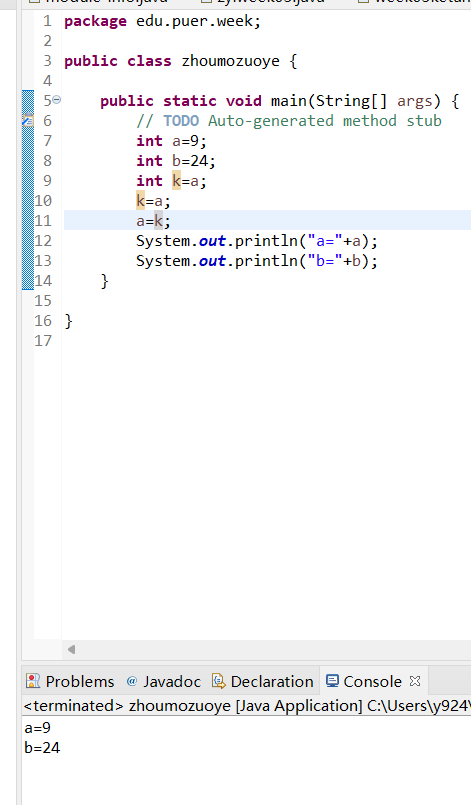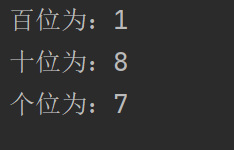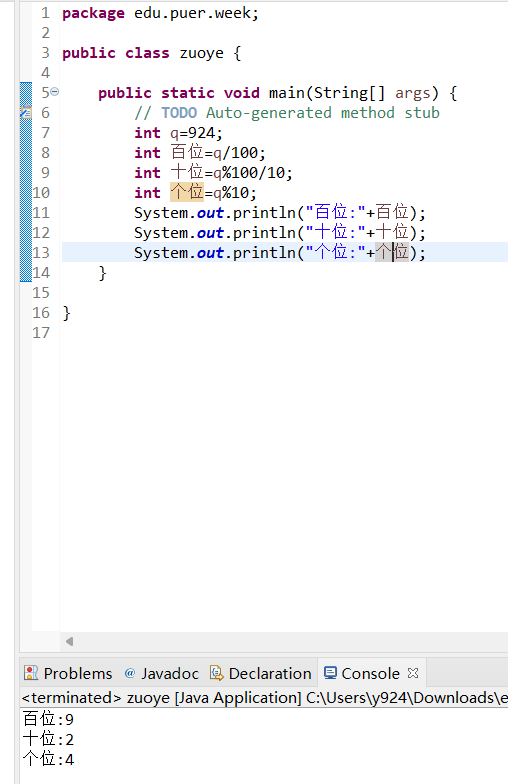# 1903021125 第三周 彭仕妹 JAVA-表达式运算

 项目 内容 课程班级博客链接 19级信计班（本） 这个作业要求链接 java第三周作业 博客名称 1903021125—彭仕妹—Java第三周作业—表达式运算 要求 每道题要有题目,代码(使用插入代码,不会插入代码的自己查资料解决，不要直接截图代码！！),截图（只截运行结果）。
• 题目1：

• 定义三个整型变量a、b、c，他们的初始值分别为4、15、20。对表达式进行运算。
• public class SuanShuDemo {
• public static void main(String[] args) {
• //声明三个整型变量a、b、c
• //分别为a,b,c赋值为4,15,20
• //计算(b/a)+c的值 ，并赋值给变量m
• //计算(c%b)*a-c的值，并赋值给变量n
• //输出m和n的值 } }

package edu.puer.zyl;

public class week03ketang {
public static void main(String[] args) {
int a;
int b;
int c;
int m,n;
a=4;
b=15;
c=20;
m=(b/a)+c;
n=(c%b)*a-c;
System.out.println("m="+m);
System.out.println("n="+n);
}
}• 题目2：

• 已知a,b均是整型变量，写出将a,b两个变量中的值互换的程序。（知识点：变量和运算符综合应用）

package edu.puer.week;

public class zhoumozuoye {

public static void main(String[] args) {
// TODO Auto-generated method stub
int a=9;
int b=24;
int k=a;
k=a;
a=k;
System.out.println("a="+a);
System.out.println("b="+b);
}

}• 题目3：

• 随意给出一个整数，打印显示他的个位数，十位数，百位数的值。（知识点：取余、除）
• 输出样例：package edu.puer.week;

public class zuoye {

public static void main(String[] args) {
// TODO Auto-generated method stub
int q=924;
int 百位=q/100;
int 十位=q%100/10;
int 个位=q%10;
System.out.println("百位:"+百位);
System.out.println("十位:"+十位);
System.out.println("个位:"+个位);
}

}• 题目4：

• 把摄氏温度37度转换为华氏温度， 摄氏温标（C）和华氏温标（F）之间的换算关系为：F=C×1.8+32 C=(F-32)÷1.8

package edu.puer.zyl;

public class week03ketang {
public static void main(String[] args) {
double x;
double y;
x=37;
y=x*1.8+32;
x=(y-32)/1.8;
System.out.println("x="+x);
System.out.println("y="+y);
}
}eclipse创建java程序，首先创建一个新的java project，取好名字后在project下创建一个package(包)，同样需要重新取名字，在packge下创建一个新的class，创建class时需要勾选主函数，所有创建完成后便可以直接编写代码。

java语言基础包括基本结构、标识符和关键字、基本数据类型、变量与常量、代码注释，一个完整的代码通常由上述内容构成。标识符由字母、数字、下划线和美元符号组成，第一个字符不能为数字，并且区分大小写，不能为关键字或保留字。当局部变量与成员变量名字相同时，此时成员变量将被隐藏，即这个成员变量在此方法中暂时失效。

posted @ 2022-03-19 23:44  怪侠一头猪  阅读(24)  评论(0编辑  收藏  举报